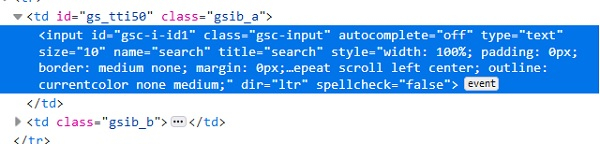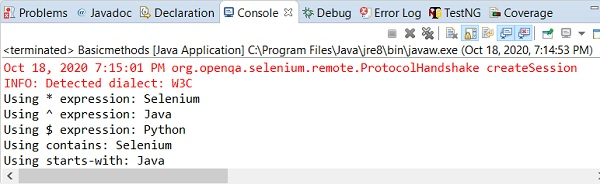# How to locate element by partial id match in Selenium?

We can locate elements by partial id match with Selenium webdriver. This can be achieved while we identify elements with the help of xpath or css locator. With the css and xpath expression, we use the regular expression to match id partially.

Let us have a look at the id of an element in its html code. The id attribute value is gsc-i-id1.With the css expression, we can use the * and perform a partial match with the id.The css value shall be input[id*='id']. This means the subtext id is present in the actual text gsc-i-id1. We can also use the ^ and perform a match with the id. The css value shall be input[id^='gsc']. This means the actual text gsc-i-id1 starts with the subtext gsc. We can also use the $and perform a match with the id. The css value shall be input[id$='id1']. This means the actual text gsc-i-id1 ends with the subtext id1.

With the xpath expression, we can use the contains() method and perform a partial match with the id. The xpath value shall be //*[contains(@id, 'id')]. This means the subtext id is present in the actual text gsc-i-id1. . We can also use the starts-with() and perform a match with the id. The css value shall be //*[starts-with(@id, 'gsc')]. This means the actual text gsc-i-id1 starts with the subtext gsc. We can also use the ends-with() and perform a match with the id. The css value shall be //*[endswith(@id, 'id1')]. This means the actual text gsc-i-id1 ends with the subtext id1.

## Example

Code Implementation.

import org.openqa.selenium.WebDriver;
import org.openqa.selenium.chrome.ChromeDriver;
import org.openqa.selenium.WebElement;
import org.openqa.selenium.By;
public class PartialMatchId{
public static void main(String[] args) {
System.setProperty("webdriver.chrome.driver", "C:\Users\ghs6kor\Desktop\Java\chromedriver.exe");
WebDriver driver = new ChromeDriver();
// identify element with partial id match with * in css
WebElement l=driver.findElement(By.cssSelector("input[id*='id']"));
l.sendKeys("Selenium");
// obtain the value entered with getAttribute method
System.out.println("Using * expression: " +l.getAttribute("value"));
l.clear();
// identify element with partial id match with ^ in css
WebElement m=driver.findElement(By.cssSelector("input[id^='gsc']"));
m.sendKeys("Java");
// obtain the value entered with getAttribute method
System.out.println("Using ^ expression: " +m.getAttribute("value"));
m.clear();
// identify element with partial id match with $in css WebElement n = driver.findElement(By.cssSelector("input[id$='id1']"));
n.sendKeys("Python");
// obtain the value entered with getAttribute method
System.out.println("Using \$ expression: " +n.getAttribute("value"));
n.clear();
// identify element with partial id match with contains in xpath
WebElement o=driver.findElement(By.xpath("//input[contains(@id,'id')]"));
o.sendKeys("Selenium");
// obtain the value entered with getAttribute method
System.out.println("Using contains: " +o.getAttribute("value"));
o.clear();
// identify element with partial id match with starts-with in xpath
WebElement p=driver.findElement(By.xpath("//input[starts-with(@id,'gsc')]"));
p.sendKeys("Java");
// obtain the value entered with getAttribute method
System.out.println("Using starts-with: " +p.getAttribute("value"));
p.clear();
driver.close();
}
}

## Output